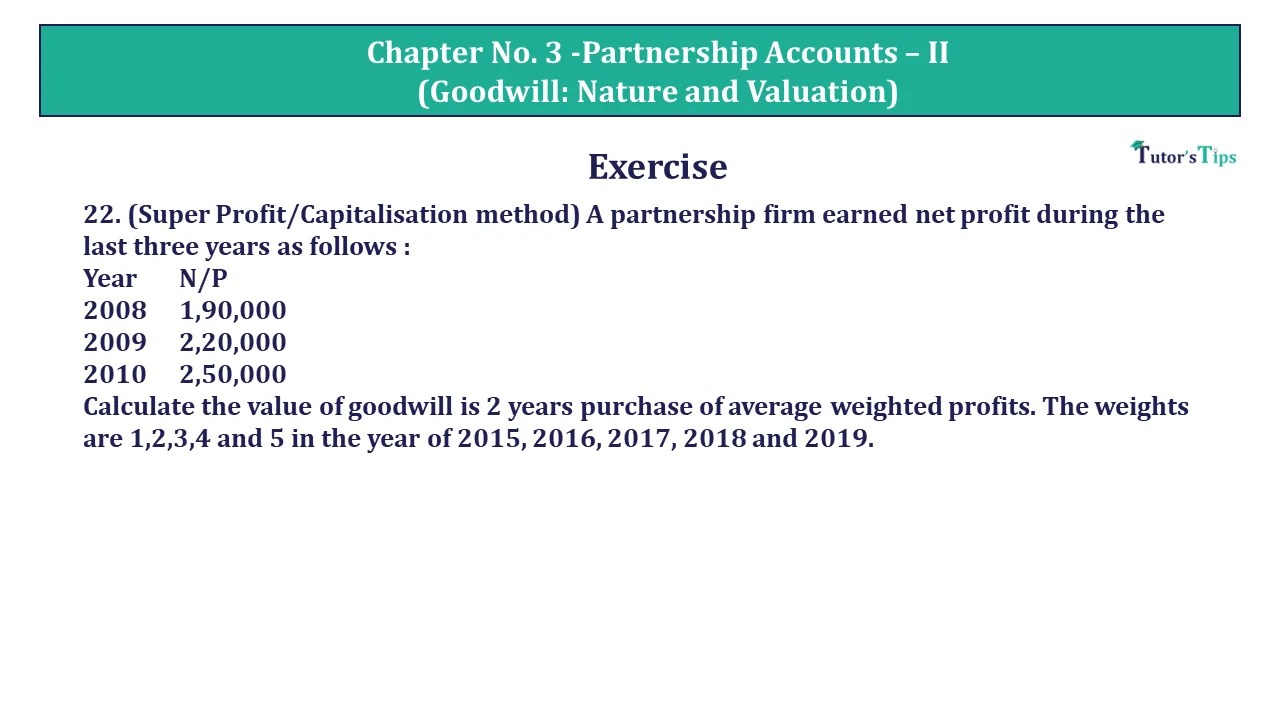# Question 22 Chapter 3 of USHA Publication 12 Class Part – 1Question 22 Chapter 3 of +2 Part-1 - USHA Publication 12 Class Part - 1

Question 22 Chapter 3 of USHA Publication 12 Class Part – 1

22. (Super Profit Method) A partnership firm earned net profit during the last three years as follows :

 Year N/P 2008 1,90,000 2009 2,20,000 2010 2,50,000

Calculate the value of goodwill is 2 years purchase of average weighted profits. The weights are 1,2,3,4 and 5 in the year of 2015, 2016, 2017, 2018 and 2019.

## The solution of Question 22 Chapter 3 of USHA Publication 12 Class Part – 1: –

 Year N/P 2008 1,90,000 2009 2,20,000 2010 2,50,000
 Average Adjusted profit = Total adjusted profit No. of year purchases = 1,20,000

 Normal Profit = Capital Employed X Normal Rate of Return 100 = 4,00,000 X 15 100 = 60,000

 Calculation of Super Profit = Average Adjusted Profit – Normal Profit = 1,20,000  – 60,000 = 60,000 Calculation of Super Profit = Average Adjusted Profit – Normal Profit Goodwill = 1,20,000 – 60,000 Goodwill = 60,000 Capitalised value of the Goodwill = Super Profits x Number of years’ purchase = 60,000 x 2 = 1,20,000
 ii) Capitalisation of Business : Goodwill = Average Profit X 100 Normal Rate of Return = 1,20,000 X 100 15 = 8,00,000
 Goodwill = Capitalised value of the business – Average Capital = 8,00,000 – 4,00,000 = 4,00,000

Comment if you have any questions.

Also, Check out the solved question of previous Chapters: –